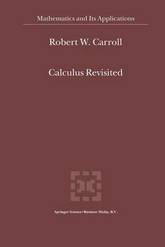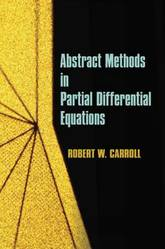#### Robert W. Carroll author

Image credit: dr. shordzi, on Flickr
Filter
(found 4 products)In this book the details of many calculations are provided for access to work in quantum groups, algebraic differential calculus, noncommutative geometry, fuzzy physics, discrete geometry, gauge theory, quantum integrable systems, braiding, finite topological spaces, some aspects of geometry and quantum mechanics and gravity.
Calculus Revisited
In this book the details of many calculations are provided for access to work in quantum groups, algebraic differential calculus, noncommutative geometry, fuzzy physics, discrete geometry, gauge theory, quantum integrable systems, braiding, finite topological spaces, some aspects of geometry and quantum mechanics and gravity.
230.990000 USD

## Calculus Revisited

by R.W. Carroll
HardbackAbstract Methods in Partial Differential Equations